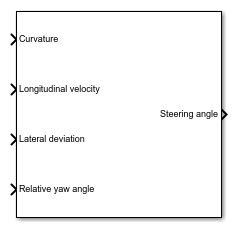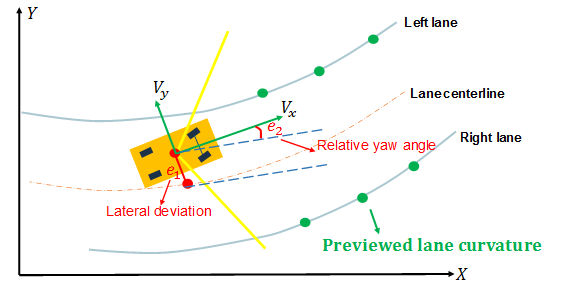# Lane Keeping Assist System

Simulate lane-keeping assistance using adaptive model predictive controller

• Library:
• Model Predictive Control Toolbox / Automated Driving

•## Description

The Lane Keeping Assist System block simulates a lane keeping assist (LKA) system that keeps an ego vehicle traveling along the center of a straight or curved road by adjusting the front steering angle. The controller reduces the lateral deviation and relative yaw angle of the ego vehicle with respect to the lane centerline. The block computes optimal control actions while satisfying steering angle constraints using adaptive model predictive control (MPC).To customize your controller, for example to use advanced MPC features or modify controller initial conditions, click .

## Ports

### Input

expand all

Road curvature, specified as 1/R, where R is the radius of the curve in meters.

• Positive when the road curves toward the positive Y axis of the global coordinate system.

• Negative when the road curves toward the negative Y axis of the global coordinate system.

• Zero for a straight road.

The controller models the road curvature as a measured disturbance with previewing. You can specify the curvature as a:

• Scalar signal — Specify the curvature for the current control interval. The controller uses this curvature value across the prediction horizon.

• Vector signal with length less than or equal to the Prediction Horizon — Specify the current and predicted curvature values across the prediction horizon. If the length of the vector is less than the prediction horizon, then the controller uses the final curvature value in the vector for the remainder of the prediction horizon.

Ego vehicle velocity in m/s.

Ego vehicle lateral deviation in meters from the centerline of the lane.

Ego vehicle longitudinal axis angle in radians from the centerline of the lane.

Minimum front steering angle constraint in radians. Use this input port when the minimum steering angle varies at run time.

#### Dependencies

To enable this port, select Use external source for the Minimum steering angle parameter.

Maximum front steering angle constraint in radians. Use this input port when the maximum steering angle varies at run time.

#### Dependencies

To enable this port, select Use external source for the Maximum steering angle parameter.

Controller optimization enable signal. When this signal is:

• Nonzero, the controller performs optimization calculations and generates a Steering angle control signal.

• Zero, the controller does not perform optimization calculations. In this case, the Steering angle output signal remains at the value it had when the optimization was disabled. The controller continues to update its internal state estimates.

#### Dependencies

To enable this port, select the Use external signal to enable or disable optimization parameter.

Actual steering angle in radians applied to the ego vehicle. The controller uses this signal to estimate the ego vehicle model states. Use this input port when the control signal applied to the ego vehicle does not match the optimal control signal computed by the model predictive controller. This mismatch can occur when, for example:

• The Lane Keeping Assist System is not the active controller. Maintaining an accurate state estimate when the controller is not active prevents bumps in the control signal when the controller becomes active.

• The steering actuator fails and does not provide the correct control signal to the ego vehicle.

#### Dependencies

To enable this port, select the Use external control signal for bumpless transfer between PFC and other controllers parameter.

State matrix of ego vehicle predictive model. The number of rows in the state matrix corresponds to the number of states in the predictive model. This matrix must be square.

The ego vehicle predictive model defined by Vehicle dynamics matrix A, Vehicle dynamics matrix B, and Vehicle dynamics matrix C must be minimal.

#### Dependencies

To enable this port, select the Use vehicle model parameter.

Input-to-state matrix of ego vehicle predictive model. The number of rows in this signal must match the number of rows in Vehicle dynamics matrix A.

The ego vehicle predictive model defined by Vehicle dynamics matrix A, Vehicle dynamics matrix B, and Vehicle dynamics matrix C must be minimal.

#### Dependencies

To enable this port, select the Use vehicle model parameter.

State-to-output matrix of ego vehicle predictive model. The number of columns in this signal must match the number of rows in Vehicle dynamics matrix A.

The ego vehicle predictive model defined by Vehicle dynamics matrix A, Vehicle dynamics matrix B, and Vehicle dynamics matrix C must be minimal.

#### Dependencies

To enable this port, select the Use vehicle model parameter.

### Output

expand all

Front steering angle control signal in radians generated by the controller. The front steering angle is the angle of the front tires from the longitudinal axis of the vehicle. The steering angle is positive towards the positive lateral axis of the ego vehicle.

## Parameters

expand all

### Parameters Tab

#### Ego Vehicle

Select this parameter to define the ego vehicle model used by the MPC controller by specifying properties of the ego vehicle. The ego vehicle model is the linear model from the front steering angle to the lateral velocity and yaw angle rate. For more information, see Ego Vehicle Predictive Model.

To define the vehicle model, specify the following block parameters:

• Total mass

• Yaw moment of inertia

• Longitudinal distance from center of gravity to front tires

• Longitudinal distance from center of gravity to rear tires

• Cornering stiffness of front tires

• Cornering stiffness of rear tires

For more information on the ego vehicle model, see Ego Vehicle Predictive Model.

Selecting this parameter clears the Use vehicle model parameter.

Select this parameter to define the state-space matrices of the ego vehicle model used by the MPC controller. This model is the linear model from the front steering angle in radians to the lateral velocity in meters per second and yaw angle rate in radians per second. For more information on the ego vehicle model, see Ego Vehicle Predictive Model.

To define the initial internal model, specify the A, B, and C state-space matrices. The internal model must be a minimal realization with no direct feedthrough, and the dimensions of A, B, and C must be consistent.

Typically, the ego vehicle steering model is velocity-dependent, and therefore, it varies over time. To update the internal model at run time, use the Vehicle dynamics A, Vehicle dynamics B, and Vehicle dynamics C input ports.

Selecting this parameter clears the Use vehicle parameters parameter.

Ego vehicle mass in kg.

#### Dependencies

To enable this parameter, select the Use vehicle parameters parameter.

Moment of inertia about the ego vehicle vertical axis in mNs2.

#### Dependencies

To enable this parameter, select the Use vehicle parameters parameter.

Distance from the ego vehicle center of mass to its front tires in meters, measured along the longitudinal axis of the vehicle.

#### Dependencies

To enable this parameter, select the Use vehicle parameters parameter.

Distance from the ego vehicle center of mass to its rear tires in meters, measured along the longitudinal axis of the vehicle.

#### Dependencies

To enable this parameter, select the Use vehicle parameters parameter.

Front tire stiffness in N/rad, defined as the relationship between the side force on the front tires and the angle of the tires to the longitudinal axis of the vehicle.

#### Dependencies

To enable this parameter, select the Use vehicle parameters parameter.

Rear tire stiffness in N/rad, defined as the relationship between the side force on the rear tires and the angle of the tires to the longitudinal axis of the vehicle.

#### Dependencies

To enable this parameter, select the Use vehicle parameters parameter.

Initial state matrix of ego vehicle predictive model. The number of rows in the state matrix corresponds to the number of states in the predictive model. This matrix must be square.

The initial ego vehicle predictive model defined by A, B, and C must be minimal.

Typically, the ego vehicle model varies over time. To update the state matrix at run time, use the Vehicle dynamics A input port.

#### Dependencies

To enable this parameter, select the Use vehicle model parameter.

Initial input-to-state matrix of ego vehicle predictive model. The number of rows in this parameter must match the number of rows in A.

The initial ego vehicle predictive model defined by A, B, and C must be minimal.

Typically, the ego vehicle model varies over time. To update the input-to-state matrix at run time, use the Vehicle dynamics B input port.

#### Dependencies

To enable this parameter, select the Use vehicle model parameter.

Initial state-to-output matrix of ego vehicle predictive model. The number of columns in this parameter must match the number of rows in A.

The initial ego vehicle predictive model defined by A, B, and C must be minimal.

Typically, the ego vehicle model varies over time. To update the state-to-output matrix at run time, use the Vehicle dynamics C input port.

#### Dependencies

To enable this parameter, select the Use vehicle model parameter.

Initial velocity of the ego vehicle model when the lane-keeping assist is enabled in m/s. This velocity can differ from the actual ego vehicle initial velocity.

### Note

A very small initial velocity, for example `eps`, can produce a nonminimal realization for the controller plant model, causing an error. To prevent this error, set the initial velocity to a larger value, for example `1e-3`.

Total transport lag, τ, in the ego vehicle model in seconds. This lag includes actuator, sensor, and communication lags. For each input-output channel, the transport lag is approximated by:

`$\frac{1}{\tau s+1}$`

#### Lane Keeping Controller Constraints

Minimum front steering angle constraint in radians.

If the minimum steering angle varies over time, add the Minimum steering angle input port to the block by selecting Use external source.

#### Dependencies

This parameter must be less than the Maximum steering angle parameter.

Maximum front steering angle constraint in radians.

If the maximum steering angle varies over time, add the Maximum steering angle input port to the block by selecting Use external source.

#### Dependencies

This parameter must be greater than the Minimum steering angle parameter.

#### Model Predictive Controller Settings

Controller sample time in seconds.

Controller prediction horizon steps. The controller prediction time is the product of the sample time and the prediction horizon.

Closed-loop controller performance. The default parameter value provides a balanced controller design. Specifying a:

• Smaller value produces a more robust controller with smoother control actions.

• Larger value produces a more aggressive controller with a faster response time.

When you modify this parameter, the change is applied to the controller immediately.

### Block Tab

Configure the controller to apply a suboptimal solution after a specified maximum number of iterations, which guarantees the worst-case execution time for your controller.

#### Dependencies

After selecting this parameter, specify the Maximum iteration number parameter.

Maximum number of controller optimization iterations.

#### Dependencies

To enable this parameter, select the Use suboptimal solution parameter.

To add the Enable optimization input port to the block, select this parameter.

To add the External control signal input port to the block, select this parameter.

Generate a custom LKA subsystem, which you can modify for your application. The controller configuration data for the custom controller is exported to the MATLAB® workspace as a structure.

You can modify the custom controller subsystem to:

• Modify default MPC settings or use advanced MPC features.

• Modify the default controller initial conditions.

expand all